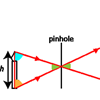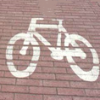# Search by Topic

#### Resources tagged with Similarity and congruence similar to Pythagoras Proofs:

Filter by: Content type:
Age range:
Challenge level:

### There are 46 results

Broad Topics > Angles, Polygons, and Geometrical Proof > Similarity and congruence### Pythagoras Proofs

##### Age 14 to 16 Challenge Level:

Can you make sense of these three proofs of Pythagoras' Theorem?### Parallel Universe

##### Age 14 to 16 Challenge Level:

An equilateral triangle is constructed on BC. A line QD is drawn, where Q is the midpoint of AC. Prove that AB // QD.### Angle Trisection

##### Age 14 to 16 Challenge Level:

It is impossible to trisect an angle using only ruler and compasses but it can be done using a carpenter's square.### Matter of Scale

##### Age 14 to 16 Challenge Level:

Prove Pythagoras' Theorem using enlargements and scale factors.### Folding Fractions

##### Age 14 to 16 Challenge Level:

What fractions can you divide the diagonal of a square into by simple folding?### Nicely Similar

##### Age 14 to 16 Challenge Level:

If the hypotenuse (base) length is 100cm and if an extra line splits the base into 36cm and 64cm parts, what were the side lengths for the original right-angled triangle?### Same Length

##### Age 11 to 16 Challenge Level:

Construct two equilateral triangles on a straight line. There are two lengths that look the same - can you prove it?### Folding Squares

##### Age 14 to 16 Challenge Level:

The diagonal of a square intersects the line joining one of the unused corners to the midpoint of the opposite side. What do you notice about the line segments produced?### Kite in a Square

##### Age 14 to 16 Challenge Level:

Can you make sense of the three methods to work out the area of the kite in the square?### Fitting In

##### Age 14 to 16 Challenge Level:

The largest square which fits into a circle is ABCD and EFGH is a square with G and H on the line CD and E and F on the circumference of the circle. Show that AB = 5EF. Similarly the largest. . . .### Sitting Pretty

##### Age 14 to 16 Challenge Level:

A circle of radius r touches two sides of a right angled triangle, sides x and y, and has its centre on the hypotenuse. Can you prove the formula linking x, y and r?### How Big?

##### Age 11 to 14 Challenge Level:

If the sides of the triangle in the diagram are 3, 4 and 5, what is the area of the shaded square?### Pent

##### Age 14 to 18 Challenge Level:

The diagram shows a regular pentagon with sides of unit length. Find all the angles in the diagram. Prove that the quadrilateral shown in red is a rhombus.### Hex

##### Age 11 to 14 Challenge Level:

Explain how the thirteen pieces making up the regular hexagon shown in the diagram can be re-assembled to form three smaller regular hexagons congruent to each other.### Fit for Photocopying

##### Age 14 to 16 Challenge Level:

Explore the relationships between different paper sizes.### Tennis

##### Age 11 to 14 Challenge Level:

A tennis ball is served from directly above the baseline (assume the ball travels in a straight line). What is the minimum height that the ball can be hit at to ensure it lands in the service area?### Pentakite

##### Age 14 to 18 Challenge Level:

ABCDE is a regular pentagon of side length one unit. BC produced meets ED produced at F. Show that triangle CDF is congruent to triangle EDB. Find the length of BE.### Partly Circles

##### Age 14 to 16 Challenge Level:

What is the same and what is different about these circle questions? What connections can you make?### Overlap

##### Age 11 to 14 Challenge Level:

A red square and a blue square overlap so that the corner of the red square rests on the centre of the blue square. Show that, whatever the orientation of the red square, it covers a quarter of the. . . .### Doubly Isosceles

##### Age 14 to 16 Short Challenge Level:

Find the missing distance in this diagram with two isosceles triangles### Triangular Tantaliser

##### Age 11 to 14 Challenge Level:

Draw all the possible distinct triangles on a 4 x 4 dotty grid. Convince me that you have all possible triangles.### All Tied Up

##### Age 14 to 16 Challenge Level:

A ribbon runs around a box so that it makes a complete loop with two parallel pieces of ribbon on the top. How long will the ribbon be?### Mapping the Wandering Circle

##### Age 14 to 16 Challenge Level:

In the diagram the point P can move to different places around the dotted circle. Each position P takes will fix a corresponding position for P'. As P moves around on that circle what will P' do?### The Line and Its Strange Pair

##### Age 14 to 16 Challenge Level:

In the diagram the point P' can move to different places along the dotted line. Each position P' takes will fix a corresponding position for P. If P' moves along a straight line what does P do ?### Pinhole Camera

##### Age 11 to 14 Challenge Level:

Make your own pinhole camera for safe observation of the sun, and find out how it works.### Squirty

##### Age 14 to 16 Challenge Level:

Using a ruler, pencil and compasses only, it is possible to construct a square inside any triangle so that all four vertices touch the sides of the triangle.### Napkin

##### Age 14 to 16 Challenge Level:

A napkin is folded so that a corner coincides with the midpoint of an opposite edge . Investigate the three triangles formed .### Slippage

##### Age 14 to 16 Challenge Level:

A ladder 3m long rests against a wall with one end a short distance from its base. Between the wall and the base of a ladder is a garden storage box 1m tall and 1m high. What is the maximum distance. . . .### Figure of Eight

##### Age 14 to 16 Challenge Level:

On a nine-point pegboard a band is stretched over 4 pegs in a "figure of 8" arrangement. How many different "figure of 8" arrangements can be made ?### From All Corners

##### Age 14 to 16 Challenge Level:

Straight lines are drawn from each corner of a square to the mid points of the opposite sides. Express the area of the octagon that is formed at the centre as a fraction of the area of the square.### Arrh!

##### Age 14 to 16 Challenge Level:

Triangle ABC is equilateral. D, the midpoint of BC, is the centre of the semi-circle whose radius is R which touches AB and AC, as well as a smaller circle with radius r which also touches AB and AC. . . .### Triangular Slope

##### Age 14 to 16 Short Challenge Level:

Can you find the gradients of the lines that form a triangle?### Triangle in a Triangle

##### Age 14 to 16 Challenge Level:

Can you work out the fraction of the original triangle that is covered by the inner triangle?### A Sameness Surely

##### Age 14 to 16 Challenge Level:

Triangle ABC has a right angle at C. ACRS and CBPQ are squares. ST and PU are perpendicular to AB produced. Show that ST + PU = AB### It Depends on Your Point of View!

##### Age 14 to 16 Challenge Level:

Anamorphic art is used to create intriguing illusions - can you work out how it is done?### Wedge on Wedge

##### Age 14 to 16 Challenge Level:

Two right-angled triangles are connected together as part of a structure. An object is dropped from the top of the green triangle where does it pass the base of the blue triangle?### Making Sixty

##### Age 14 to 16 Challenge Level:

Why does this fold create an angle of sixty degrees?### Points in Pairs

##### Age 14 to 16 Challenge Level:

In the diagram the radius length is 10 units, OP is 8 units and OQ is 6 units. If the distance PQ is 5 units what is the distance P'Q' ?### A Shade Crossed

##### Age 14 to 16 Challenge Level:

Find the area of the shaded region created by the two overlapping triangles in terms of a and b?### Trapezium Four

##### Age 14 to 16 Challenge Level:

The diagonals of a trapezium divide it into four parts. Can you create a trapezium where three of those parts are equal in area?### Look Before You Leap

##### Age 14 to 16 Challenge Level:

Can you spot a cunning way to work out the missing length?### Triangle Midpoints

##### Age 14 to 16 Challenge Level:

You are only given the three midpoints of the sides of a triangle. How can you construct the original triangle?##### Age 14 to 16 Challenge Level:

Two ladders are propped up against facing walls. The end of the first ladder is 10 metres above the foot of the first wall. The end of the second ladder is 5 metres above the foot of the second. . . .### Two Triangles in a Square

##### Age 14 to 16 Challenge Level:

Given that ABCD is a square, M is the mid point of AD and CP is perpendicular to MB with P on MB, prove DP = DC.### Bus Stop

##### Age 14 to 16 Challenge Level:

Two buses leave at the same time from two towns Shipton and Veston on the same long road, travelling towards each other. At each mile along the road are milestones. The buses' speeds are constant. . . .